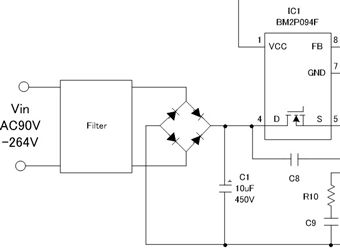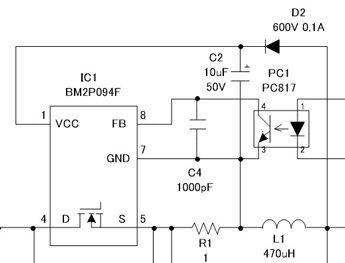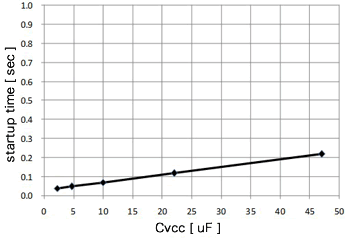Technical Information Site of Power Supply Design

• Basic Knowledge
• AC/DC
• Design Examples of Non-isolated Buck Converters
• Selecting Critical Components: Input Capacitor C1 and VCC Capacitor C2

2017.10.12 AC/DC

# Selecting Critical Components: Input Capacitor C1 and VCC Capacitor C2

Design Examples of Non-isolated Buck Converters

In the previous section, the IC to be used in design was determined, and so we immediately move on to selection of external components and calculation of component values.

Input capacitor: C1

The capacitor C1 is connected to an input line that uses a bridge to rectify the input AC voltage.

The capacitance of the input capacitor can be determined using the following table as a guideline.

 Input voltage (ＶAC) Cin (µF) 85-264 2×Pout(W) 180-264 1×Pout(W)In this example, the input voltage range is 90 VAC to 264 VAC, and so 2 x Pout is used. However, the figures in the table are for full-wave rectification, and adjustments may be necessary when conditions are different or depending on the specification value for the input voltage hold time.

Pout is determined from the output specification. The output is 20 V/0.2 A, and so the capacitance of C1 is determined as follows.

Pout = 20 V×0.2 A = 4 W
C1 = 2×4 = 8　⇒　10 µF is used

Next, the capacitor’s rated voltage is determined. As is clear from the circuit diagram, the input voltage, that is, the voltage applied to this capacitor, is the voltage resulting from rectification of the maximum input voltage, or 1.41 times VAC (max).

For 264 VAC:
264 V×1.41 = 372 V　⇒　a voltage rating of 400 V or higher is used

In the circuit of this example, an operating margin is considered in selecting a capacitor with a voltage rating of 450 V.

Capacitor for VCC: C2

Next, we determine capacitor C2 for VCC. The VCC capacitor is necessary to stabilize the VCC voltage of the power supply IC generated from the output.

The datasheet for the power supply IC recommends a capacitance for C2 of 2.2 µF or higher. Considering the output voltage, a value of 50 V/10 µF is selected.

Further, C2 also has the role of determining the startup time for the IC when power is turned on. The relationship between the capacitance of C2 and the startup time is indicated in the graph shown below, which appears in the data sheet. The startup time for a capacitance of 10 µF is approximately 0.08 sec. If it is necessary to adjust the startup time, another capacitance of 2.2 µF or higher, such as 22 µF, can be selected.#### Key Points:

・The rated voltage of the input capacitor is selected considering that a voltage equal to 1.41 times the maximum input voltage is applied.

・It should be remembered that in addition to stabilizing VCC, the VCC capacitor also determines the startup time.